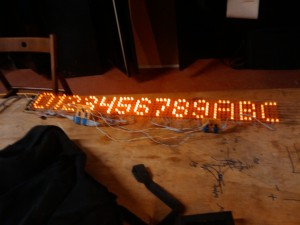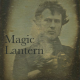It’s been a while since we had a March Madness post, so here’s a little snippet from this past weekend: a script for processing small pixel fonts for use in 8-bit AVR applications.  Like this:I couldn’t find any free 7-pixel-high fonts that I liked, so I whipped one up in GIMP.  Here’s the source image that I generated the font from:Getting a raw B&W image into a usable format after the cut.

The 8-bit AVRs have very little memory, so I stored each 7-pixel-high column as a single byte.  The pixel data, along with the table that maps ASCII codes to character data, comes in at around 500 bytes.  I’m storing the data in program space, which is where the goofy avr/pgmspace.h stuff comes in.

Here’s the python script. It takes a single parameter– the name of the image file. It generates a header and source file that contain the font data, along with a couple of helpful functions for getting the pixels of a character and its size.

```#!/usr/bin/env python

from PIL import Image
from optparse import OptionParser
from string import Template
import sys

# pngToFont takes a simple 1-bit image of a pixel font
# and converts it to a chunk of C code that we can use
# in microcontroller code.

# Edit this to reflect the order that characters appear
# in your font.  Each character should be seperated by
# one column of white pixels.
char_order = "ABCDEFGHIJKLMNOPQRSTUVWXYZ0123456789.!?@/:;()"

# Header and types for font.
# We're a little tight on space, so it's program memory
# for you.  Hooray for Harvard architectures.
#include
#include

typedef struct {
uint8_t offset;
uint8_t len;
} PROGMEM char_entry;

#define FONT_HEIGHT \$height
#define FONT_DATA_SIZE \$data_size

extern char_entry font_table;
extern prog_uint8_t font_data[FONT_DATA_SIZE];

uint8_t get_char_len(uint8_t c);
uint8_t get_char_bit(uint8_t c, uint8_t row, uint8_t column);

""")

font_source_templ = Template("""

char_entry font_table = {
\$font_table
};

prog_uint8_t font_data[FONT_DATA_SIZE] = {
\$font_data
};

uint8_t get_char_len(uint8_t c) {
}

uint8_t get_char_bit(uint8_t c, uint8_t row, uint8_t column) {
return ((col & _BV(row)) == 0)?0:1;
}

""")

def get_column(im,x_offset):
"Get the bitmap column as an int, with black pixels as 1"
(_, height) = im.size
val = 0
for bit in range(height):
if im.getpixel((x_offset,bit)) == 0:
val = val | (1 << bit)
return val

class Character:
def __init__(self,im,x_offset):
self.len = 0
self.columns = []
column = get_column(im,x_offset)
while column != 0:
self.columns.append(column)
self.len = self.len + 1
x_offset = x_offset + 1
column = get_column(im,x_offset)

charmap = {}

def png_to_font(im,c_path,h_path):
c_file = open(c_path,"w")
h_file = open(h_path,"w")
x_offset = 0
data_size = 0
# load all the characters from the image file
for char in char_order:
charmap[char] = Character(im,x_offset)
x_offset = x_offset + charmap[char].len + 1
data_size = data_size + charmap[char].len
print "Char " + char + " is len " + str(charmap[char].len)
count=len(char_order),
data_size=data_size,
height=im.size))
h_file.close
# build the tables
font_data = ""
font_table = ""
offset = 0
for i in range(128):
char = chr(i)
if charmap.has_key(char):
c = charmap[char]
c.offset = offset
font_table = font_table + "  {%d, %d},\n" % (offset,c.len)
font_data = font_data + ",\n".join(map(hex,c.columns)) + ",\n"
offset = offset + c.len
else:
font_table = font_table + "  {0,0},\n"
# write out the source
c_file.write(font_source_templ.substitute(
font_table = font_table,
font_data = font_data,
c_file.close()

def main():
parser = OptionParser(usage="usage: %prog [options] source")
help="output to given base path")
(options,args) = parser.parse_args()
if len(args) != 1:
parser.error("Please provide a single input file.")
image = Image.open(args)
image = image.convert("L")
c_path = "font.c"
h_path = "font.h"
if (options.out_path):
c_path = options.out_path + ".c"
h_path = options.out_path + ".h"
png_to_font(image,c_path,h_path)

if __name__ == "__main__":
main()
```

### 4 Responses to “March Madness Backlog: Microcontroller Pixel Font”

1.I wrote a similar set of fonts for my Canon 5D project. mkfont parses the fonts into packed 8, 16 or 32-bit wide rows. At the time I didn't have a multiply instruction, so there is some funky math going on in the display routines.

This is the font-small.

•Neat! Looks like we've been implementing parallel code. Alas, your 8-pixel-high font is one pixel too high for my purposes. 🙂

•I generated the fonts with bigtext, which can translate X11 fonts into arbitrary sizes. The font-*.in files are output via generate-font script.

2.how about a instructable on how to make an led sign?